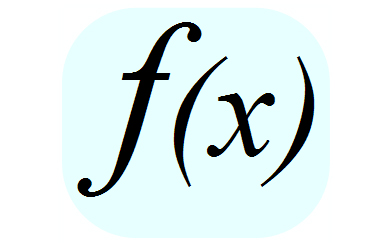# Fun With Functions

Algebra Level 2If $f(-4)=1 \text{ and } g(x)=f(\frac{x}{-3})$, what point can you determine on the graph of g?

×